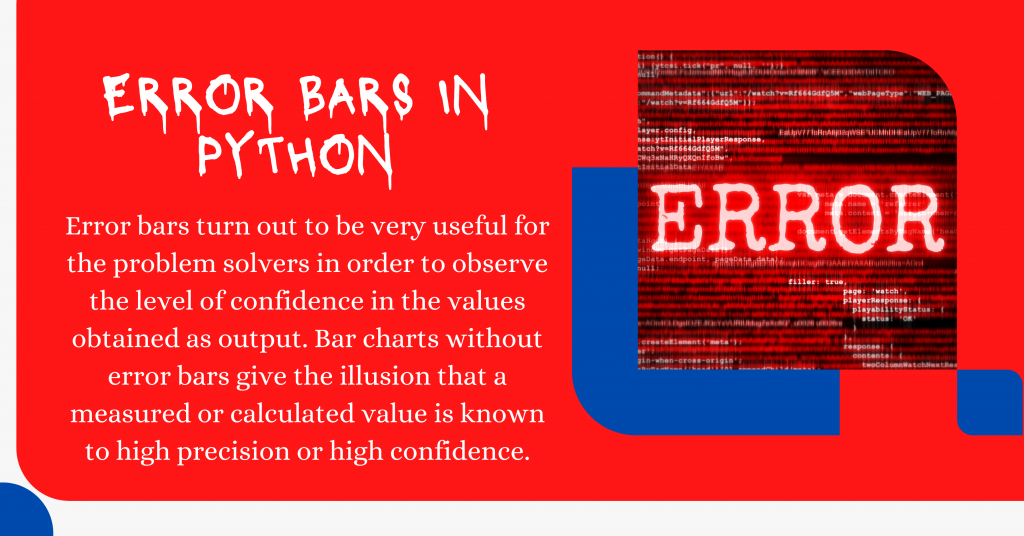# Introduction to Error Bars in PythonToday in this tutorial, we will understand the concept of error bars and learn how to visualize the same in the Python programming language.

Let me first introduce you to errorbars before jumping to the code.

## What are Error Bars in Python?

In data science studies, displaying error bars in your visualizations is inevitable and is much needed.

Error bars turn out to be very useful for the problem solvers in order to observe the level of confidence in the values obtained as output. Bar charts without error bars give the illusion that a measured or calculated value is known to have high precision or high confidence.

## Implementing Error Bars in Python

Now that we know what error bars are let’s learn to visualize them using basic machine learning modules.

### 1. Importing Modules

```import numpy as np
import matplotlib.pyplot as plt
%matplotlib inline
plt.style.use('seaborn')
```

### 2. Visualizing Error bars in Bar Plots

In order to visualize error bars in Bar plots, we have to create the bar plot first. The same is done by creating the data and plot the bar plot first.

#### 2.1 Data Creation

To create data, we will be taking 3 different objects. Here, for this tutorial, we will be representing the thermal temperature of three metals.

```aluminum = np.array([6.4e-5 , 3.01e-5 , 2.36e-5, 3.0e-5, 7.0e-5, 4.5e-5, 3.8e-5,
4.2e-5, 2.62e-5, 3.6e-5])
copper = np.array([4.5e-5 , 1.97e-5 , 1.6e-5, 1.97e-5, 4.0e-5, 2.4e-5, 1.9e-5,
2.41e-5 , 1.85e-5, 3.3e-5 ])
steel = np.array([3.3e-5 , 1.2e-5 , 0.9e-5, 1.2e-5, 1.3e-5, 1.6e-5, 1.4e-5,
1.58e-5, 1.32e-5 , 2.1e-5])
```

Visualization plays a major role in any ML program, so, let us visualize the data in a scatter plot using the code mentioned below.

```x_values = np.array([i for i in range(len(aluminum))])
plt.scatter(x_values,aluminum,label="Aluminium")
plt.scatter(x_values,copper,label="Copper")
plt.scatter(x_values,steel,label="Steel")
plt.title("Initial Data Visualization")
plt.legend()
plt.show()
```

#### 2.2 Calculate Error for Error bars

In order to get errors for errorbars, we require the mean and the standard deviation which can be computed with the help of the mean and the std the function of numpy array.

The code for the same is displayed below. We will also be preparing this data for plotting by creating a few variables:

1. List of lables
2. List containing mean of each category
3. List containing standard deviation of each category
```aluminum_mean = np.mean(aluminum)
copper_mean = np.mean(copper)
steel_mean = np.mean(steel)

aluminum_std = np.std(aluminum)
copper_std = np.std(copper)
steel_std = np.std(steel)

labels = ['Aluminum', 'Copper', 'Steel']
x_pos = np.arange(len(labels))
CTEs = [aluminum_mean, copper_mean, steel_mean]
error = [aluminum_std, copper_std, steel_std]
```

#### 2.3 Plotting Error Bars

The code to plot the error bars is mentioned below where the error is the standard deviation of the values in each category.

```plt.style.use('seaborn')
fig, ax = plt.subplots()
ax.bar(x_pos, CTEs,
yerr=error,
align='center',
alpha=0.2,
color='green',
ecolor='red',
capsize=10)

ax.set_ylabel('Coefficient of Thermal Expansion')
ax.set_xticks(x_pos)
ax.set_xticklabels(labels)
ax.set_title('Coefficent of Thermal Expansion (CTE) of Three Metals')
ax.yaxis.grid(True)
plt.show()
```

### 3. Visualizing Error bars in Line Plots

Error bars can also be added to line plots created with Matplotlib.

The `ax.errorbar()` method is used to create a line plot with error bars which requires lists or arrays of x, y data points.

The code for the same is displayed below.

```x = np.linspace(0,5.5,5)
y = np.exp(-x)

xerr = np.random.random_sample(5)
yerr = np.random.random_sample(5)
fig, ax = plt.subplots()

ax.errorbar(x, y,
xerr=xerr,
yerr=yerr,
fmt='-o',
color='yellow',
ecolor='green')

ax.set_xlabel('x-axis')
ax.set_ylabel('y-axis')
ax.set_title('Line plot with error bars')
plt.show()
```